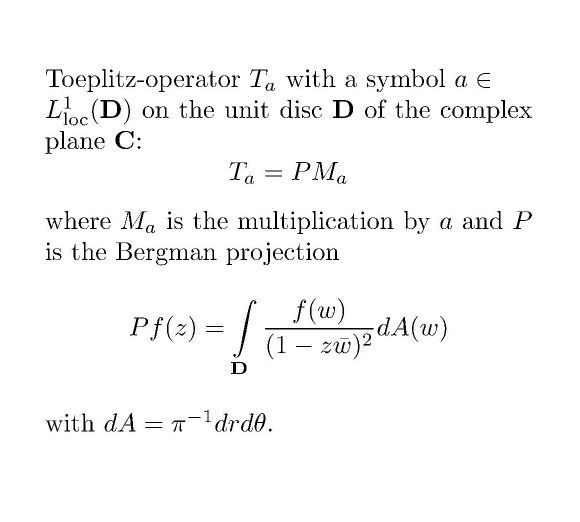Main page Department of Mathematics and Statistics of University of HelsinkiFormula 1. Toeplitz-operator and Bergman projection Toeplitz-operators Ta considered here are defined as the compose of the multiplication Ma and the standard unweighted orthogonal Bergman projection P. SOME RECENT ARTICLES J.Bonet, J.Taskinen: Toeplitz-operators on the space of analytic functions with logarithmic growth. J.Math.Anal.Appl. 353 (2009), 428-435. The second author introduced the inductive limit spaces H and L as a replacement of the standard Banach spaces of bounded analytic (respectively, measurable) functions on the unit disc, due to the reason that the Bergman projection P: L → H is a continuous operator, contrary to the case of the original Banach spaces. In the present article we give a characterization of positive symbols a such that the corresponding Toeplitz-operators Ta : H → H are continuous or compact. The conditions are quite natural growth conditions for the Berezin symbol of a, much in the spirit of Zhu's charaterization of bounded Toeplitz-operators on the Bergman-Hilbert-space. This gives some further evidence that the space H should be considered at least as a good "associate member" in the family of reflexive Bergman spaces! For general nonpositive symbols we also give sufficient condition for continuity and compactness. M.Engliš, J.Taskinen: Deformation quantization and Borel's theorem in locally convex spaces. Studia Math. 180,1 (2007), 77-93. This paper deals with deformation quantization, where Toeplitz operators can sometimes be used to construct star products (so called Berezin-Toeplitz quantization for example on bounded symmetric domains). We contribute to the conjecture that every star product is induced by Hilbert space operators, by showing that this is true once the star product is equivalent to the Toeplitz star product. The main technical tool is Colombeau's generalization of Borel's theorem, on Taylor coefficients of analytic functions, to the case of Fréchet-space valued analytic functions. Back to main page .